# Chocholate pyramid

How many chocolates are in the third shelf when at the 8th shelf are 41 chocolates in any other shelf is 7 chocolates more the previous shelf.

Correct result:

x =  6

#### Solution:

a+7d = 41
d = 7
x = a+2d

a+7•d = 41
d = 7
x = a+2•d

a+7d = 41
d = 7
a+2d-x = 0

a = -8
d = 7
x = 6

Our linear equations calculator calculates it.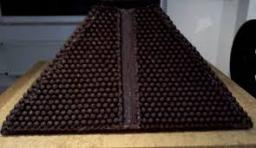We would be pleased if you find an error in the word problem, spelling mistakes, or inaccuracies and send it to us. Thank you!Tips to related online calculators
Do you have a linear equation or system of equations and looking for its solution? Or do you have quadratic equation?

## Next similar math problems:

• Arithmetic progression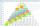In some AP applies: 5a2 + 7a5 = 90 s3 = 12 Find the first member a =? and difference d = ?
• Three ints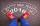The sum of three consecutive integers is 2016. What numbers are they?
• Chairs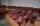Determine the number of seats in the seventh row and ninth row, if 3rd row has 14 seats and in every next row of seats has five more than the previous row.
• Bonuses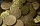Five employees of the company were paid bonuses so that each successor received a 550 USD less than the previous employee. How much did everyone get, if a total of USD 11,000 has paid?
• GP - three membersThe second and third of a geometric progression are 24 and 12(c+1) respectively, given that the sum of the first three terms of progression is 76 determine value of c
• RectanglePerimeter of rectangle is 48 cm. Calculate its dimensions if they are in the ratio 5:3 (width:height)
• Determine AP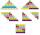Determine the difference of the arithmetic progression if a3 = 7, and a4 + a5 = 71
• Hexagon cut pyramidCalculate the volume of a regular 6-sided cut pyramid if the bottom edge is 30 cm, the top edge us 12 cm, and the side edge length is 41 cm.
• Fifth memberDetermine the fifth member of the arithmetic progression, if the sum of the second and fifth members equal to 73, and difference d = 7.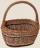Determine how many apples are in baskets when in the first basket are 4 apples, and in any other is 29 apples more than the previous, and we have eight baskets.
• Height of pyramidThe pyramid ABCDV has edge lengths: AB = 4, AV = 7. What is its height?
• The regularThe regular quadrilateral pyramid has a volume of 24 dm3 and a height of 45 cm. Calculate its surface.
• Geometric seqFind the third member of geometric progression if a1 + a2 = 36 and a1 + a3 = 90. Calculate its quotient.
• Shelf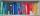How many ways are there to arrange 6 books on a shelf?
• Rectangular triangleThe lengths of the rectangular triangle sides with a longer leg of 12 cm form an arithmetic sequence. What is the area of the triangle?
• Tetrahedron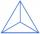What is the angle of the sides from the base of a three-sided pyramid where the sides are identical?
• In the 8In the A. P. 36, 39, 42, …, which term is 276?